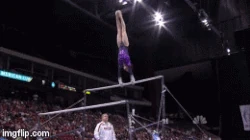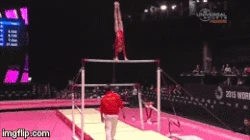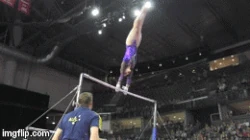On uneven bars, balance beam, and floor exercise, the connection of two or more elements earn extra tenths of bonus towards the routine's start value. Depending on how difficult the connected skills are, they have different connection values, or CV. If there is too long of a pause between the elements in a planned connection, it's not credited and causes the start value to drop. These connection values are as listed in the 2022-2024 Code of Points.

Values

On bars, beam, and floor, each element is given a value, which represents how difficult it is. Values are represented as letters (A-H on bars and beam; A-J on floor), which correspond to how many tenths they're worth.

A = 0.1
B = 0.2
C = 0.3
D = 0.4
E = 0.5
F = 0.6
G = 0.7
H = 0.8
I = 0.9
J = 1.0

Uneven Bars

For flight elements, they must be directly connected, meaning no kip cast in between.

Connection & Value Example 1 Example 2
D + D (or more) = +0.1 CVpiked Stalder with half turn (D) + piked Jaeger (E)Healy (E) + Ling (E)
D + E (both being flight
elements) = +0.1 CVRicna (E) + Geinger (D)Komova (E) + Pak (D)
D (flight element/transition
from LB to HB) + C (or more,
performed on HB) = +0.2 CV
(must be performed in this
order)Tkatchev (D) + full turn (C)Komova II (E) + Stalder full turn (D)
E + E (or more, at least
one being a flight
element) = +0.2 CVpiked Stalder full (E) + Komova (E)Nabieva (F) + Bhardwaj (E)
F + D (or more,
both being flight
elements) = +0.2 CVTweddle (F) + Ezhova (D)Downie (F) + Pak (D)

Balance Beam

Acrobatic Connections

Connection & Value Example 1 Example 2
C + C = +0.1 CVlayout step-out (C) + layout step-out (C)layout step-out (C) + back pike (C)
B + D = +0.1 CVback handspring (B) + Onodi (D)back handspring (B) + Rulfova (D)
B + E = +0.1 CVround-off (B) + layout (E)back handspring (B) + layout (E)
C/D + D (or more, with
rebound) = +0.2 CVside aerial (D) + layout step-out (C)Kochetkova (D) + layout step-out (C)
B + D (rebound forward) =
+0.2 CVfront handspring (B) + front pike (D)free aerial (D) + front tuck (D)
B + F = +0.2 CVback handspring (B) + tucked full (F)back handspring (B) + Arabian (F)
B + F (or more, dismount) =
+0.2 CVround-off (B) + triple twist dismount (F)round-off (B) + double Arabian dismount (G)
Starting from B + B + C
(any order; can include
mount or dismount) =
+0.1 SB (series bonus)back handspring (B) + layout step-out (C) +
layout step-out (C)round-off (B) + back handspring (B) +
double Arabian dismount (G)

​Elements with rebound go in the same direction, elements without rebound go in different directions.

Dance & Mixed Connections

Connection & Value Example 1 Example 2
C + C (or more, both being
dance elements) = +0.1 CVswitch leap (C) + switch leap half (D)switch leap (C) + Johnson (C)
A + C (both being turns) =
+0.1 CVL turn (C) + full turn (A)double turn (D) + full turn (A)
D + B (mixed) = +0.1 CVfree aerial (D) + split jump (B)switch ring leap (E) + back handspring (B)
D (salto to 1 foot) + A Scale =
+0.1 CVfree aerial (D) + scale (A)side aerial (D) + scale (A)
D + D (or more) = +0.2 CVOnodi (D) + illusion (D)switch half (D) + Onodi (D)
Starting from B + B + C
(any order) = +0.1 SB
(series bonus)switch leap (C) + switch half (D) + back pike (C)free aerial (D) + split jump (B) + back handspring (B)

Floor Exercise

Indirect Acrobatic Connections

Indirect connections are when the first skill is separated by a round-off and/ or back handspring from the second skill, but the entire sequence is connected together.

Connection & Value Example 1 Example 2
B/C + D = +0.1 CVbackward 1½ twist (C) + 2½ twist (D)front layout (B) + double tuck (D)
A + A + D =  +0.1 CVwhip (A) + whip (A) + double tuck (D)whip (A) + whip (A) + 2½ twist (D)
C + E = +0.2 CVbackward 1½ twist (C) + double Arabian
(E)backward 1½ twist (C) + triple twist (E)
D + D = +0.2 CV2½ twist (D) + double tuck (D)2½ twist (D) + triple twist (E)
A + A + E = +0.2 CVwhip (A) + whip (A) + full-twisting double
back (E)whip (A) + whip (A) + triple twist (E)

Direct Acrobatic Connections

Connection & Value Example 1 Example 2
A + D = +0.1 CVwhip (A) + double pike (D)2½ twist (D) + front tuck (A)
C + C = +0.1 CVbackward 1½ twist (C) + forward 1½
twist (C)front layout full (C) + forward 1½ twist
(C)
D + B = +0.1 CV2½ twist (D) + front layout step-out (B)front layout (B) + front double twist (D)
A + E = +0.2 CVwhip (A) + triple twist (E)double Arabian (E) + front tuck (A)
C + D = +0.2 CV2½ twist (D) + front layout full (C)front layout full (C) + front double full (D)

Mixed Connections

Connection & Value Example 1 Example 2
D (salto) + B (dance) = +0.1 CVdouble pike (D) + sheep jump (B)​​​
double pike (D) + split jump ½ (B)
E (salto) + A (dance) = +0.1 CVdouble Arabian (E) + stag jump (A)double layout (F) + wolf jump (A)

Turns Connections

Connection & Value Example 1 Example 2
D + B = +0.1 CVdouble turn in back attitude (D) + double
turn (B)Memmel turn (D) + illusion (B)